# Fuzzy Logic Model for Optimal Operation of Darbandikhan Reservoir, Iraq

Fuzzy Logic Model for Optimal Operation of Darbandikhan Reservoir, Iraq

Mariwan R. FarisHekmat M. Ibrahim Kawa Z. Abdulrahman Luqman S. Othman Kilgour D. Marc

Civil Engineering Department, College of Engineering, University of Kirkuk, Kirkuk 36001, Iraq

Water Resources Engineering Department, College of Engineering, University of Sulaimani, Sulaimani 46001, Iraq

Civil Engineering Department, University of Halabja, Halabja 46018, Iraq

Wilfrid Laurier University, Waterloo N2L 3C5, Canada

Corresponding Author Email:
mrnfaris63@uokirkuk.edu.iq
Page:
335-343
|
DOI:
https://doi.org/10.18280/ijdne.160401
28 June 2021
|
Accepted:
10 August 2021
|
Published:
31 August 2021
| Citation

OPEN ACCESS

Abstract:

The operating system of a reservoir is a significant contributor to its success in water resources management. For a multi-purpose reservoir, an ideal operating system determines output characteristics that take account of all objectives. In this study, a new model to optimize Darbandikhan reservoir system operation in Iraq is proposed, based on a fuzzy logic controller. The aim of the new system is to maximize hydropower production while satisfying all downstream water demands. The proposal was tested on flow and other data in 324 monthly time steps, from January 1992 to December 2018, using a simulation model based on the Simulink technique of MATLAB software. Trial and error showed that membership functions of the Gaussian shape were most appropriate. For comparison purposes, Discrete Differential Dynamic Programming (DDDP), Nonlinear Programming (NLP) and Linear Programming (LP) optimization models were developed and applied to operate the reservoir. The results for turbine operation show that, relative to actual power produced, average monthly hydropower generation is increased by 32.2% (fuzzy logic), 51.4% (DDDP), 29.3% (NLP), 27.4% (LP). Although the dynamic programming method generated more hydropower, the fuzzy logic controller avoided complex optimization procedures, making it more flexible and more acceptable to operators. Moreover, the fuzzy approach provides an opportunity for reservoir operators to develop and apply more convenient operating rules, which in itself is a valuable alternative to conventional optimization techniques.

Keywords:

dynamic programming, fuzzy logic controller, hydropower generation, reservoir operation, simulation model

1. Introduction

The operating system of a reservoir specifies the amount of stored and released water. Many techniques have been developed to optimize reservoir system operation such as; Discrete Differential Dynamic Programming (DDDP), Nonlinear Programming (NLP), Linear Programming (LP), Genetic Algorithm (GA). Comparative models for reservoir hydropower generation displayed that DDDP provide better results of hydropower production than GA and NLP . However, some researchers discussed the limitations and complexity of these optimization models and the necessity of using more flexible and expert based knowledge approaches (such as Fuzzy Logic) in reservoir operation modelling [2, 3]. In recent studies of ambiguity, modeling has focused on fuzzy logic-based approaches to regulate water resources systems [4, 5]. Tilmant et al.  made a comparison study between reservoir operating policies obtained from fuzzy and nonfuzzy explicit stochastic dynamic programming. They showed that both approaches yield similar measures of system performance. On the other hand, several Fuzzy rule-based models were developed to optimize the operation of single and multi-purpose reservoirs [4, 7-9]. Mehta & Jain  developed an operation policy for a multi-purpose reservoir using the Neuro-Fuzzy technique. The developed operation policy was applied to reduce the damage due to floods, droughts, and determines optimum releases against demands for domestic water supply, irrigation, and hydropower generation for monsoon and non-monsoon periods.

Some researchers focused on fuzzy logic and stochastic programming to derive optimal operating policies for the hydropower reservoir system and solution of hydropower problems within the reservoir system [3, 11, 12]. Also, several studies were conducted for developing models to derive the optimal operating policies for single and multi-reservoir systems during flood and drought periods based on the combination of fuzzy logic with other techniques such as linear programming, dynamic programming, conventional hedging rule, and genetic algorithm [13-17].

Furthermore, several efforts were made to modify the performance of Fuzzy logic models by developing new algorithms, tools, and techniques. Esogbue & Liu  suggested a new tool of fuzzy criterion decision processes for the treatment of complex reservoir operation problems. Akbari et al.  developed a new method to predict river flow using a fuzzy rule-based model with a modified algorithm which improves the performance of the model as new data becomes accessible. Fu  presented a novel fuzzy optimization method for multi-criteria decision-making problems under a fuzzy environment. Refaey  proposed a new technique for automatically manufacture of fuzzy membership functions and fuzzy rules from a set of data points.

The present study aims to derive an optimal operating policy to maximize the hydropower generation against downstream demands (agricultural, domestic, industrial, and environmental) for Darbandikhan Reservoir System (DRS) in Kurdistan Region, Iraq. For this purpose, a Fuzzy Logic Controller (FLC) based reservoir operation model is developed for a multi-purpose reservoir. In addition, for comparison purpose, DDDP, NLP and LP optimization methods have been developed for the DRS operation. The study proposes an opportunity to change conventional reservoir operation to optimized methodologies in order to develop optimized operation rule curves. Thus, it is necessary to create awareness of the usefulness of the modern method to solve water resource problems.

The paper is organized into four main sections following this introduction. Section 2 presents the materials and methods adopted in this study. Section 3 presents the results obtained from different optimization models and discuss their performances. Finally, the paper presents conclusions in Section 4.

2. Materials and Methods

The present study is an endeavor to improve a mechanism for managing the operational issues in Darbandikhan reservoir. To optimize the reservoir operation, an appropriate technique was selected using a simple fuzzy logic-based approach. For comparison purposes, the foremost commonly utilized procedures for optimizing reservoir operation, were developed. The fundamental and principle theories of these techniques related to reservoir optimization, including data and information processing, objectives, and constraints identification that has been adopted in the present study, are described in the following sections.

2.1 Study area and data preparation

2.1.1 Study area

The current study is carried out on the Darbandikhan reservoir located on the Diyala river approximately 230 km Northeast of Baghdad and 65 km South-East of Sulaimani city, Kurdistan Region, Iraq as shown in Figure 1. It was built between 1956 and 1961 with a total storage capacity of 3.0 milliard cubic meters. The dam is rock filled type with impervious clay core and rockfill shoulders. The characteristics of Darbandikhan dam and reservoir are presented in Table 1.

## 1.png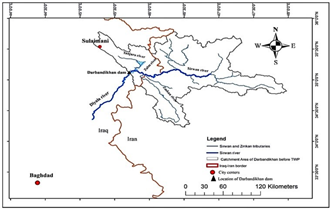Figure 1. Location of the Darbandikhan dam and reservoir on Diyala river (adopted from )

Table 1. Characteristics of Darbandikhan dam and reservoir (Adopted from Darbandikhan Dam Directorate)

 Items Characteristics Crest level (lowest) El. 495.0 m ASL Maximum height 128.0 m Crest length (including spillway width) 535.0 m Width of crest 17.0 m Normal operation level El. 485.0 m ASL Minimum drawdown level El. 434.0 m ASL Live storage volume at El. 485.0 2,500 Mm³ Dead storage volume 500 Mm³

A hydropower station with a maximum capacity of 249 MW was installed in 1992. It consists of 3 x 83 MW Francis-type turbines. Furthermore, the reservoir is controlled by a gated spillway structure found on the right bank. It comprises three 15 m x 15 m corrupted entryways introduced on an ogee shape molded flood segment taken after a steep chute ending in a redirector bucket. Dividing walls partitioned the releases from each of the three-door openings. The most extreme release capacity of the spillway at ordinary working level (El. 485.00m ASL) is 5,700 m³/s and 11,400 m³/s at an estimated height of flood level (El. 493.50m ASL) .

2.1.2 Data preparation

The available data used for developing the fuzzy models in the current study are the mean monthly inflows to the Darbandikhan reservoir and the actual monthly releases over the period, January 1992 to December 2018, i.e. (324) mean monthly inflow and releases. Figure 2 shows the mean monthly inflow data to the Darbandikhan reservoir for the 324 months. The inflow data of Darbandikhan dam are obtained by operation balance, and hence the losses are implicitly included. The maximum and minimum values of inflow discharges are 861.31 m3/s and 2.16 m3/s in April 1992 and August 2000, respectively. At the same time, the average monthly multiannual data is about 101.5 m3/s.

Water conveyance for different sectors (domestic, agriculture, industrial, environmental and so on) is essential for reservoirs. The water released from Darbandikhan dam provides water for different projects located between Darbandikhan dam and Hemrein dam. The main projects are Shaik Langer, Balajo Khanaqin, Qara Tappe, Jalawla, and Al-Saadia . Also, it is the main source of water supply for the domestic use for Kalar district. The environmental water requirement is also determined based on the historically recorded minimum water release for the downstream of the reservoir. Table 2 displays the total monthly water requirement for downstream of Darbandikhan reservoir.

## 2.pngFigure 2. Monthly inflow discharges into Darbandikhan reservoir for the period Jan 1992 to Dec 2018

Table 2. Downstream water demands for Darbandikhan reservoir (Adopted from )

 Month Jan. Feb. Mar. Apr. Downstream demand (Mm3) 41.60 59.60 81.60 111.40 Month May Jun. Jul. Aug. Downstream demand (Mm3) 93.40 105.40 108.00 95.00 Month Sep. Oct. Nov. Dec. Downstream demand (Mm3) 94.00 110.40 88.40 46.40

Another aim of Darbandikhan reservoir is to protect the downstream areas from flooding. During the flood season (December to April), the storage level in the reservoir should be lowered below 485 m. a. s. l. according to a previously prepared flood control operation rule, as shown in Figure 3.

## 3.png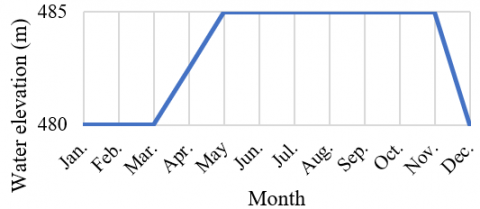Figure 3. Control levels for Darbandikhan reservoir (Adopted from Darbendikhan Dam Directorate)

2.2 Formulated fuzzy logic approach

The successful applications of fuzzy logic and fuzzy sets can be attributed to the fact that the fuzzy model imitates the true situation of the real world, where human thinking is dominated by approximate reasoning logic. FLC is a rule based system that incorporates the flexibility of human decision making by means of the use of the fuzzy set theory .

In numerous cases, fuzzy logic may give the foremost suitable technique for modeling reservoir operation. For illustration, in a reservoir operation problem, the storages and the inflows may shape the premises, and the discharge may characterize the consequences. Based on expert supposition or from the information of the chronicled operation, the rules can be developed .

The membership functions (MFs) play a crucial part in the general execution of fuzzy representation. The shapes of MFs are critical for a specific problem since they influence a fuzzy inference framework. They may have distinctive shapes such as triangular, trapezoidal, Gaussian and so forth. The trial and error strategy is regularly utilized for MFs shape since there is no exact strategy for choosing the MFs . Mamdani fuzzy inference system (FIS) is the most commonly used fuzzy technique. This technique is the first control system developed using fuzzy set theory. In this inference, the fuzzy sets from the consequent of each rule are combined through the aggregation operator to get the resulting fuzzy set. After the aggregation process, there is a fuzzy set for each output variable that needs defuzzification .

Various shapes of MFs are applied for the fuzzy models, such as Triangular, Trapezoidal and Gaussian shapes, to generate the proper output. Among the various applied shapes of MFs, the Gaussian MFs have been selected as it performed better than others. Therefore, this membership function is used in developing the proposed fuzzy model as shown is in Figure 4.

## 4.pngFigure 4. Gaussian fuzzy membership function

The function $\mu_{\mathrm{A}}$ is described as follow:

$\mu_{\mathrm{A}}(\mathrm{x})=\mathrm{e}^{-\frac{(\mathrm{x}-\mathrm{k})^{2}}{2 \sigma^{2}}}, \sigma>0$      (1)

In which $\sigma$ is the standard deviation.

2.3 Dynamic programming

Dynamic Programming (DP) is the most commonly used technique for the optimization of reservoir operation. The recursive equation of DP can be written as below .

$\mathrm{F}_{\mathrm{n}}\left(\mathrm{s}_{\mathrm{n}}\right)=\operatorname{Max}\left[\mathrm{O}_{\mathrm{n}}\left(\mathrm{s}_{\mathrm{n}}, \mathrm{d}_{\mathrm{n}}\right)+\mathrm{F}_{\mathrm{n}-1}\left(\mathrm{~S}_{\mathrm{n}-1}\right)\right]$     (2)

where, $s_{n}$ is the state variable, $d_{n}$ is the decision variable, $\mathrm{O}_{\mathrm{n}}\left(\mathrm{s}_{\mathrm{n}}, \mathrm{d}_{\mathrm{n}}\right)$ is the objective function value and $\mathrm{F}_{\mathrm{n}}\left(\mathrm{s}_{\mathrm{n}}\right)$ is the cumulative return at stage $\mathrm{n}$ with known $\mathrm{F}_{0}\left(\mathrm{~s}_{0}\right)$.

Many different versions of DP have been developed over time to alleviate the problems of dimensionality. One of them is the Discrete Differential Dynamic Programming (DDDP) . It is an iterative procedure in which the recursive condition of dynamic programming which utilized to discover a progressed direction among the discrete states within the neighborhood of a trial direction.

The present study focuses on maximizing the production of hydropower by considering downstream demand constraints. The hydropower production mainly depends on the net head, the discharge through the turbines, and the installed plant capacity . The following function is used to calculate the generated hydropower:

$H P=\frac{\gamma \cdot R \cdot H \cdot \eta}{1000}$      (3)

So, the objective function of the present study can be written as:

Maximize $H P=\sum_{i=1}^{N} \sum_{j=1}^{12} K \cdot R_{(i, j)} \cdot H_{(i, j)}$     (4)

where:

$H_{(i, j)}$ is the average available net head (m) above turbines at $\mathrm{j}^{\text {th }}$  month and $\mathrm{i}^{\text {th }}$ year.

$R_{(i, j)}$ is the discharge release (Mm3/month) through turbines in at $\mathrm{j}^{\text {th }}$ month and $\mathrm{i}^{\text {th }}$ year.

HP  is the total generated hydropower (MW).

K is a constant of the hydropower equation.

$\gamma$ is the specific weight of water (9810 N/m3).

$\eta$ is the overall efficiency of the turbines.

2.4 Nonlinear programming

Most of the reservoir operation systems include Non-linearity problems through compound relationships among numerous physical and hydrological variables. NLP techniques are utilized to solve such class of problems . In nonlinear optimization, an objective function or some of the constraints are nonlinear. Hence, getting the optimal solution is rather difficult than the linear programming. For instance, typically, hydropower generation problems, reservoir water surface area versus storage relationships are nonlinear and pose difficulties in gaining their solutions.

2.5 Linear programming

One of the most important methods for optimization is Linear programming. It is an operation approach that universally preferred and has been widely used in managing water resource planning and problems .

The objective function for maximizing hydropower production can be expressed as a product form of the release and the head for hydropower generation so nonlinear equation exists. Therefore, in this study a linear function is presented by using Taylor series theorem as an alternative objective function in place of Eq. (4) as:

Maximize $H P=\sum_{i=1}^{N} \sum_{j=1}^{12} K_{1}^{\prime} \cdot R_{(i, j)}+\sum_{i=1}^{N} \sum_{j=1}^{12} K_{2}^{\prime} \cdot H_{(i, j)}$      (5)

where, $K_{1}{ }^{\prime}$ and $K_{2}{ }^{\prime}$ are the constant values of the water release and the net head for hydropower generation, respectively. Eq. (5) can be replaced with Eq. (6) since the storage water level $H_{(i, j)}$ is directly proportional to the storage volume $S_{(i, j)}$ and the constant value $K_{1}{ }^{\prime}$ can be substituted with another constant value $K_{1}$ as:

Maximize $H P=\sum_{i=1}^{N} \sum_{j=1}^{12} K_{1} \cdot R_{(i, j)}+\sum_{i=1}^{N} \sum_{j=1}^{12} K_{2} \cdot S_{(i, j)}$     (6)

Eq. (6) is the linear combination of water release $R_{(i, j)}$ and reservoir storage $S_{(i, j)}$, and it can be represented as an alternative objective function for maximizing hydropower energy.

2.6 Reservoir operation constraints

In the optimization of reservoir operation, there are several constraints, which represent limitations on the behavior and performance of the system. These constraints are:

2.6.1 Reservoir water balance equation

The continuity equation expressed below:

$S_{(t+1)}=S_{(t)}+I_{(t)}-R_{(t)}-O_{(t)}$      (7)

where:

$S_{(t+1)}$ torage at the end of time t.

$S_{(t)}$ is the storage at the beginning of time t.

$I_{(t)}$ is the inflow discharge during the time t.

$R_{(t)}$ is the release during the time t.

$O_{(t)}$ is the overflow discharge during the time t.

Note: Any losses due to evaporation or seepage have been neglected for Darbandikhan reservoir because the inflows data are obtained by operation balance, and thus the losses are implicitly included.

2.6.2 Downstream demand constraints

Release from the reservoir must be less than or equal to the downstream demands, while these demands equal to the sum of irrigation, water supply, and other demands (municipal, industrial, and hydropower generation), which can be written as:

$R_{\min } \leq R(t) \leq R_{\max }$      (8)

where, $R_{\min }$ is the minimum discharge release required for downstream demands and $R_{\max }$ is the maximum capacity of outlet turbines.

2.6.3 Storage capacity constraints

The reservoir storage for any period should not be greater than the maximum capacity of the reservoir nor less than the dead storage of the reservoir and is expressed as:

$S_{\min } \leq S(t) \leq S_{\max }(t)$      (9)

where, $S_{\min }$ is the minimum storage of the reservoir and $S_{\max }(t)$ is the maximum capacity of the reservoir at time $t$.

2.6.4 Turbine capacity

The maximum hydropower should not be more than the turbine capacities, and this constraint can be written as:

$H P(t) \leq H P_{\max }$      (10)

in which, $\mathrm{HP}_{\max }$ is the maximum capacity of the turbines installed at the reservoir hydropower station. Also, the power plants working in a specified range of water elevation in the reservoir; therefore, the power generation is halted if the available head in the reservoir gets below the minimum head required to operate the turbines. This can be written as:

$H_{\min } \leq H(t) \leq H_{\max }$     (11)

where, $H_{\min }$ and $H_{\max }$ are the minimum and maximum net water heads required for the operation of the turbines, respectively.

2.7 Model application

To get new operational rules for Darbandikhan reservoir, FLC concept has been used. The available inflow data for Darbandikhan reservoir is based on average monthly intervals over January 1992 to December 2018 (i.e., 324) mean monthly flow records. The fundamental membership functions required by the Fuzzy Logic Algorithms were built based on the inflow and the water head in which they are representing the inputs. Hence, the inflows rate and the reservoir water elevation in each chosen simulation time step are characterized as very low, low, moderate, high, and very high separately. In comparison, the discharges have been characterized utilizing the same previously mentioned five categories to represent the output of the fuzzy controller. The selected Gaussian MFs and the corresponding rules for operating Darbandikhan reservoir are represented in Figure 5 to Figure 9 for the FLC.

The Fuzzy Logic Toolbox is designed to work seamlessly with Simulink in a MATLAB (version R2017b) environment, in this manner, the FLC has been connected to the Simulink Demonstrate. The system processes have been identified using a set of 25 rules. The inflow and elevation were given as input for the FLC model, and outflow was evaluated as the output. Also, the DDDP model has been developed by writing a MATLAB code (m-file). The solvers “fmincon” and “linprog” in optimization toolbox of MATLAB software were applied to obtain the NLP and LP models respectively. Figure 10 shows the compatibility of FLC with the Simulink model used in the current study. This applicability of this technique is illustrated for Darbandikhan reservoir operation via using 27 years of recorded average monthly inflows.

## 5.png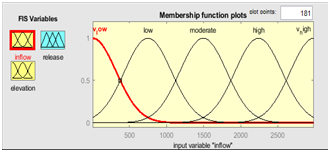Figure 5. Membership function of monthly inflow of Darbandikhan reservoir

## 6.png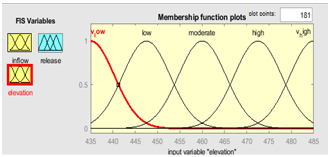Figure 6. Membership function of water elevation in Darbandikhan reservoir

## 7.png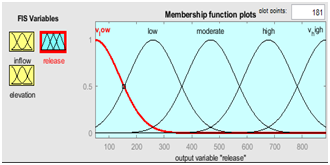Figure 7. Membership function of release (output) of Darbandikhan reservoir

## 8.pngFigure 8. Rules editor used for fuzzy controller (part of rules)

## 9.png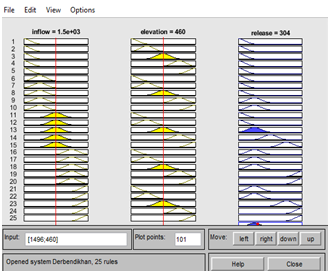Figure 9. Rules viewer used for the fuzzy controller

3. Results and Discussions

In this paper, a generic Fuzzy Inference System (FIS) has been used to optimize the Darbandikhan reservoir operation. The fuzzy rules were formulated based on the historical data recorded at the Darbandikhan dam. MATLAB (VersionR2017b) software has been used for modeling the operation of Darbandikhan reservoir using FLC. The system processes have been identified using a set of 25 rules. The inflows and elevations were given as input to the FLC model, and outflow was evaluated as the output. In addition, a comparative study based upon the results of the developed model has been carried out considering the downstream discharge release, water elevation in the reservoir and monthly hydropower generation. Implementing the established models for operating the Darbandikhan reservoir, are described in the following sections.

3.1 Hydroelectric power generation

Hydroelectric generation is one of the essential objectives of Darbandikhan reservoir. The average and monthly hydropower generated from 1992 to 2018 using the four models and the observed values are shown in Table 3, Figure 11, and Figure 12. It can be seen that the average value of the annual generated hydropower using the FLC, DDDP, NLP and LP models are 1008 MW, 1155 MW, 987 MW and 972 MW with an increase of about 32.1%, 51.3%, 29.3% and 27.4% compared to the observed generated hydropower (763 MW) respectively.

## 10.png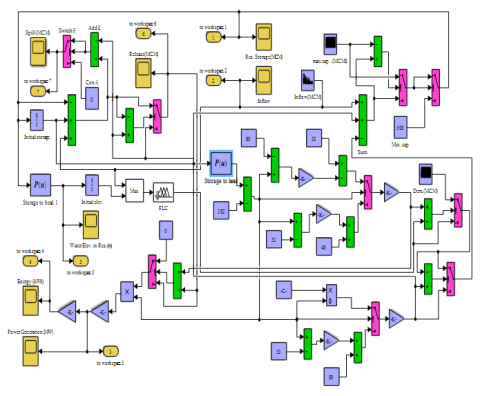Figure 10. Simulink based model for Darbandikhan reservoir with FLC imbedded within the model

## 11.png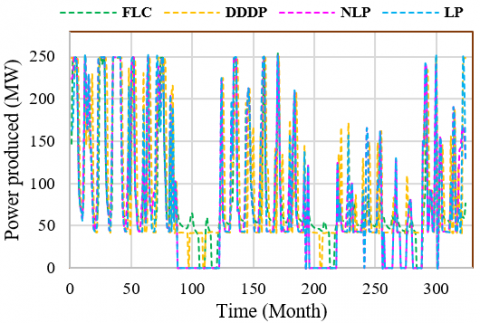Figure 11. Monthly hydropower generated by models from Darbandikhan reservoir during the operation period

The comparison of monthly hydropower generation shows increasing in hydropower generation in monsoon periods compared with other months. This is attributed to the fact that the greatest water amount is allowed to pass through the turbines during the reservoir high income discharge at monsoon periods.

## 12.png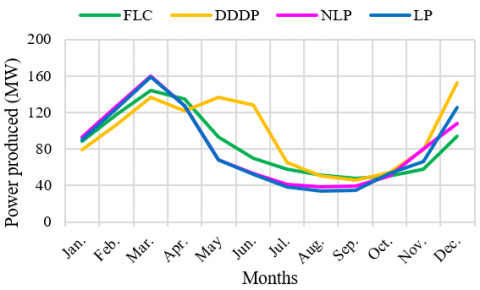Figure 12. Average monthly power produced for Darbandikhan reservoir

Table 3. Results of hydropower generated from the models

 Models Maximum monthly power generated (MW) Minimum monthly power generated (MW) Average monthly power generated (MW) FLC 248.94 0.00 84.04 DDDP 249.48 0.00 96.26 NLP 248.94 0.00 82.30 LP 248.94 0.00 81 Actual 214.20 0.00 63.58

3.2 Discharge release

Discharge release includes satisfying all water requirements for the downstream. The average water release through the turbines based on the Fuzzy logic model simulation are less than the releases based on the DDDP, NLP and LP models. Table 4, Figure 13, and Figure 14 show the discharge released through the turbines.

Table 4. Characteristics of discharge release results for the models

 Models Maximum Monthly discharge release ($\mathrm{m}^{3} / \mathrm{s}$) Minimum monthly discharge release ($\mathrm{m}^{3} / \mathrm{s}$) Average monthly discharge release ($\mathrm{m}^{3} / \mathrm{s}$) FLC 180.42 60.8 105.87 DDDP 174.34 53.31 120.72 NLP 193.29 53.76 107.78 LP 193.29 48.06 108.04

## 13.pngFigure 13. Monthly discharge release using four models for Darbandikhan reservoir during the operation period

## 14.png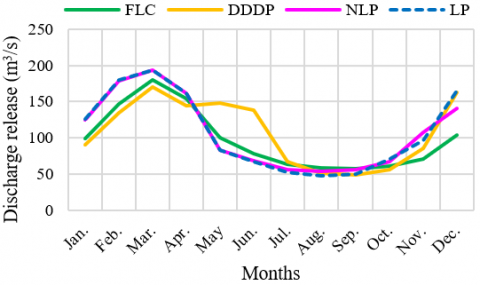Figure 14. Average monthly discharge release for Darbandikhan reservoir

The main principle in water resource management is adopting an applicable reservoir operating strategy for allocating the available water amount among different water consumers, especially during the scarcity and flood periods, and how to minimize the negative impact of both phenomenon. For these reasons, the artificial reservoirs are used as a balancing tool to regulate and balance the inflow and outflow by saving the excess amount of inflow water and releasing it in low water inflow period.

The results showed that the maximum average monthly discharge release from the reservoir during the operation period occurred in March with values of 180.42 $\mathrm{m}^{3} / \mathrm{s}$, 174.34 $\mathrm{m}^{3} / \mathrm{s}$, 193.29 $\mathrm{m}^{3} / \mathrm{s}$ and 193.29 $\mathrm{m}^{3} / \mathrm{s}$ while, the minimum average monthly discharge release occurred in August and September with values of 60.8 $\mathrm{m}^{3} / \mathrm{s}$ , 53.31 $\mathrm{m}^{3} / \mathrm{s}$, 53.76 $\mathrm{m}^{3} / \mathrm{s}$ and 48.06 $\mathrm{m}^{3} / \mathrm{s}$ according to FLC, DDDP, NLP and LP models, respectively. Also, it is worth to mention that the discharge releases with all methods for the whole considered period have satisfied all the downstream water requirements (irrigations, domestic, industrials, and environmental).

3.3 Reservoir water surface elevation

The reservoir water level variation within the (27) years of operation by implementing the Four models is presented in Table 5, Figure 15, and Figure 16. The resulting minimum average monthly water elevations are 472.23 m, 476.99 m, 475.78 m and 475.44 m. a.s.l. in December based on FLC, DDDP, NLP and LP models, respectively, while the resulting maximum elevations for the operating period were 479.44 m, 484.21 m, 483.41 m and 483.41 ma.s.l. from May to June.

## 15.png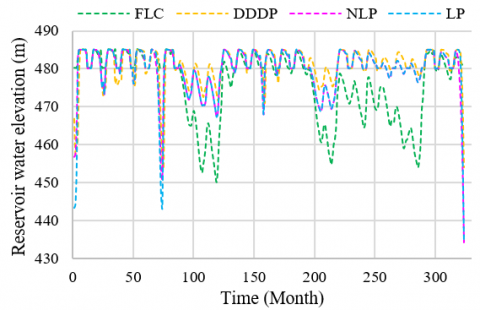Figure 15. Monthly water elevation in the Darbandikhan reservoir during the operation period

## 16.pngFigure 16. Average monthly water elevation for Darbandikhan reservoir

Table 5. Characteristics of average monthly water elevation results

 Models Maximum Monthly water elevation (m) Minimum monthly water elevation (m) Average monthly water elevation (m) FLC 479.44 472.23 475.84 DDDP 484.21 476.99 480.92 NLP 483.41 475.78 479.9 LP 483.41 475.47 479.86

It can be seen that the reservoir water elevation in the period (December to April) was not allowed to reach 485 m. a.s.l. Due to the restriction imposed by the flood control policy, it is required to keep a space to accommodate floods. Also, from the output, it has appeared that the water level during the operation period based on the DDDP, NLP and LP models are higher than FLC since the optimization algorithm searches to find the optimum path along all periods whereas, FLC is approximately determines the optimized pattern.

4. Conclusions

In the present study, the Darbandikhan reservoir operation has been optimized for improving hydropower generation using FLC, DDDP, NLP and LP models while keeping the downstream demands satisfied. The fuzzy rule-based demonstration for hydropower reservoir operation attempts to achieve an implementable hydropower reservoir operation approach. One of the main advantages of the fuzzy rule-based hydropower reservoir operation over conventional optimization methods is that the complex optimization methods are avoided.

The fuzzy rules were derived based on expert knowledge and applied to optimize the operation of the Darbandikhan reservoir. Based on the results obtained from four models for the period 1992 to 2018, it has been found that the annual generated hydropower has increased by about 32.1%, 51.3%, 29.3% and 27.4% compared to the observed generated hydropower (763 MW) using the FLC, DDDP, NLP and LP models respectively. Simultaneously, the models keep satisfying all the downstream water requirements (irrigations, domestic, industrials, and environmental) throughout the operation period.

From the results it can be figured out that FLC model performed better than NLP and LP models in producing hydropower. Moreover, even though the DDDP strategy created more hydropower than FLC, the fuzzy logic strategy is much simpler, and therefore easier to apply effectively. Hydropower reservoir operation is prone to problems when the computational burden is heavy; in comparison, an adaptable and transparent system is more likely to result in satisfactory operation in the long run. In particular, its straightforward rules will encourage hydropower reservoir administrators to take an interest in developing and augmenting the procedure. However, FLC systems have some limitations and drawbacks as it is wholly dependent on human knowledge and expertise. Also, the rules of an FLC system need to be regularly modified.

Acknowledgment

The Authors Would Like to Express Their Special Thanks of Gratitude to Darbendikhan Dam Directorate Staff, in Darbendikhan, Sulaimani, Kurdistan Region, Iraq for Their Valuable Help Throughout the Work of This Research.

References

 Othman, L.S., Ibrahim, H.M. (2017). Simulation-optimization model for dokan reservoir system operation. Sulaimania Journal for Engineering Sciences, 4(5): 7-25. https://doi.org/10.17656/sjes.10053

 Simonovic, S.P. (1992). Reservoir systems analysis: Closing gap between theory and practice. Journal of Water Resources Planning and Management, 118(3): 262-280. https://doi.org/10.1061/(ASCE)0733-9496(1992)118:3(262)

 Russell, S.O., Campbell, P.F. (1996). Reservoir operating rules with fuzzy programming. Journal of Water Resources Planning and Management, 122(3): 165-170. https://doi.org/10.1061/(ASCE)0733-9496(1996)122:3(165)

 Kamodkar, R.U., Regulwar, D.G. (2013). Multipurpose reservoir operating policies: A fully fuzzy linear programming approach. Journal of Agricultural Science and Technology, 15(6): 1261-1274.

 Moorthi, P.V.P., Singh, A.P., Agnivesh, P. (2018). Regulation of water resources systems using fuzzy logic: A case study of Amaravathi dam. Applied Water Science, 8(5): 132. https://doi.org/10.1007/s13201-018-0777-8

 Tilmant, A., Vanclooster, M., Duckstein, L., Persoons, E. (2002). Comparison of fuzzy and nonfuzzy optimal reservoir operating policies. Journal of Water Resources Planning and Management, 128(6): 390-398. https://doi.org/10.1061/(ASCE)0733-9496(2002)128:6(390)

 Shrestha, B.P., Duckstein, L., Stakhiv, E.Z. (1996). Fuzzy rule-based modeling of reservoir operation. Journal of Water Resources Planning and Management, 122(4): 262-269. https://doi.org/10.1061/(ASCE)0733-9496(1996)122:4(262)

 Panigrahi, D.P., Mujumdar, P.P. (2000). Reservoir operation modelling with fuzzy logic. Water Resources Management, 14(2): 89-109. https://doi.org/10.1023/A:1008170632582

 Soentoro, E.A., Pebriana, N. (2019). Fuzzy rule-based model to optimize outflow in single reservoir operation. MATEC Web of Conferences, 270: 04015. https://doi.org/10.1051/matecconf/201927004015

 Mehta, R., Jain, S.K. (2009). Optimal operation of a multi-purpose reservoir using neuro-fuzzy technique. Water Resources Management, 23(3): 509-529. https://doi.org/10.1007/s11269-008-9286-0

 Tilmant, A., Fortemps, P., Vanclooster, M. (2002). Effect of averaging operators in fuzzy optimization of reservoir operation. Water Resources Management, 16(1): 1-22. https://doi.org/10.1023/A:1015523901205

 Moeini, R., Afshar, A., Afshar, M.H. (2011). Fuzzy rule-based model for hydropower reservoirs operation. International Journal of Electrical Power & Energy Systems, 33(2): 171-178. https://doi.org/10.1016/j.ijepes.2010.08.012

 Balve, P.N., Patel, J.N. (2016). Optimal reservoir operation policy using fuzzy linear programming. International Journal of Advances in Mechanical and Civil Engineering, 3(4): 2394-2827.

 Mousavi, S.J., Ponnambalam, K., Karray, F. (2005). Reservoir operation using a dynamic programming fuzzy rule–based approach. Water Resources Management, 19(5): 655-672. https://doi.org/10.1007/s11269-005-3275-3

 Ahmadianfar, I., Adib, A., Taghian, M. (2016). Determination of optimal hedging rule using fuzzy set theory for multi-reservoir operation. International Journal of Optimization in Civil Engineering, 6(1): 27-42. http://ijoce.iust.ac.ir/article-1-236-en.html

 Ahmadianfar, I., Adib, A., Taghian, M. (2017). Optimization of multi-reservoir operation with a new hedging rule: Application of fuzzy set theory and NSGA-II. Applied Water Science, 7(6): 3075-3086. http://dx.doi.org/10.1007/s13201-016-0434-z

 Deka, P.C., Chandramouli, V. (2009). Fuzzy neural network modeling of reservoir operation. Water Resources Planning and Management, 135(1): 135: 5-12. https://doi.org/10.1061/(ASCE)0733-9496(2009)135:1(5)

 Esogbue, A.O., Liu, B. (2006). Reservoir operations optimization via fuzzy criterion decision processes. Fuzzy Optimization and Decision Making, 5(3): 289-305. https://doi.org/10.1007/s10700-006-0015-y

 Akbari, M., Afshar, A., Sadrabadi, M.R. (2009). Fuzzy rule based models modification by new data: application to flood flow forecasting. Water Resources Management, 23(12): 2491-2504. https://doi.org/10.1007/s11269-008-9392-z

 Fu, G. (2008). A fuzzy optimization method for multicriteria decision making: An application to reservoir flood control operation. Expert Systems with Applications, 34(1): 145-149. https://doi.org/10.1016/j.eswa.2006.08.021

 Refaey, M.A. (2016). Automatic generation of membership functions and rules in a fuzzy logic system. The Fifth International Conference on Informatics and Applications (ICIA2016), 117.

 Faraj, D.M., Zaidan, K. (2020). The impact of the tropical water project on Darbandikhan Dam and Diyala River Basin. Iraqi Journal of Civil Engineering, 14(1). https://www.iasj.net/iasj/article/183106

 World Bank. (2006). Dokan and Darbendikhan Dam Inspections. https://dokumen.tips/documents/dokan-derbendikhan-dam-inspections-world-bank-viewdokan-and-derbendikhan-dam-inspections.html

 Hussein, H.A. (2010). Dependable discharges of the upper and middle diyala basins. Journal of Engineering, 16(2): 10.

 Yang, Y., Soh, C.K. (2000). Fuzzy logic integrated genetic programming for optimization and design. Journal of Computing in Civil Engineering, 14(4): 249-254. https://doi.org/10.1061/(ASCE)0887-3801(2000)14:4(249)

 Husain, A. (2012). An overview of reservoir systems operation techniques. CiteSeerX, 4(10): 30-37.

 Heidari, M., Chow, V.T., Meredith, D.D. (1971). Water resources systems analysis of discrete differential dynamic programming. Department of Civil Engineering, University of Illinois at Urbana-Champaign, 1971.

 Loucks, D.P., Beek, E. (2017). Water Resource Systems Planning and Management: An Introduction to Methods, Models, and Applications. Springer. https://doi.org/10.1007/978-3-319-44234-1

 Rani, D., Moreira, M.M. (2010). Simulation–optimization modeling: a survey and potential application in reservoir systems operation. Water Resources Management, 24(6): 1107-1138. https://doi.org/10.1007/s11269-009-9488-0

 Raju, B.K., Chandre Gowda, C., Karthika, B.S. (2020). Optimization of reservoir operation using linear programming. International Journal of Recent Technology and Engineering (IJRTE), 8(5): 1028-1032. http://dx.doi.org/10.35940/ijrte.E6174.018520